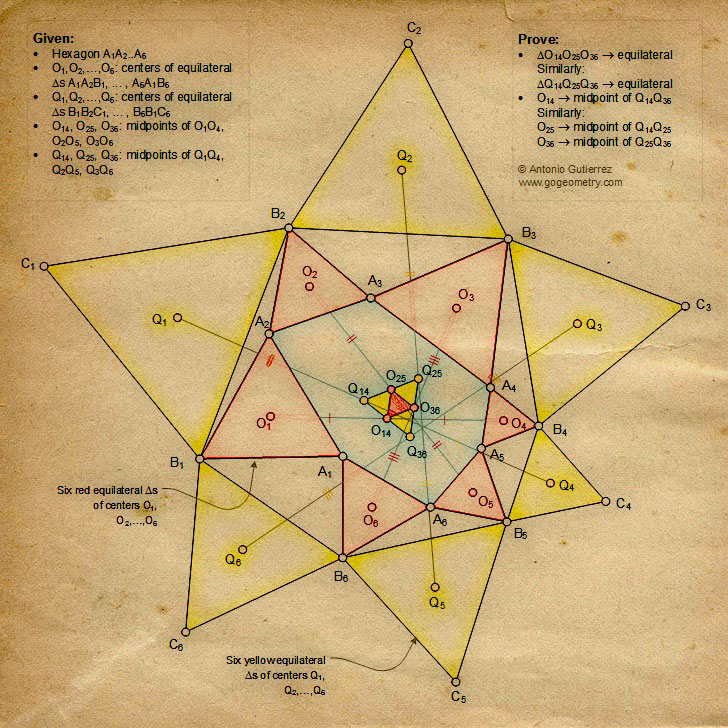# Geometric Art of Problem 1319: Hexagon, Equilateral Triangle, Midpoint, iPad Apps.

 The figure shows a hexagon A1A2A3A4A5A6 with equilateral triangles A1A2B1,... A6A1B6.of centers O1, O2, ..., O6.  Q1, Q2, ...,Q6 are the centers of equilateral triangles B1B2C1, ..., B6B1C6.  O14, O25, O36, Q14, Q25, and Q36 are the midpoints of O1O4, O2O5, O3O6, Q1Q4, Q2Q5, and Q3Q6, respectively. Prove that (1) Triangles O14O25O36 and Q14Q25Q36 are equilateral; (2) O14, O25, and O36 are the midpoints of Q14Q36, Q14Q25, and Q25Q36, respectively.Geometric Art using Mobile Apps Geometric art is a form of art based on the use and application of geometric figures. A geometric figure is any set or combination of points, lines, surfaces and solids. A mobile app or mobile application software is a computer program designed to run on smartphones and tablet computers. See also: Original problem 1319

 Home | Geometry | Problems | All Problems | Open Problems | Visual Index | 10 Problems | Problems Art Gallery | 1311-1320 | Triangle | Equilateral Triangle | Center | Hexagon | Midpoint | iPad Apps | by Antonio Gutierrez